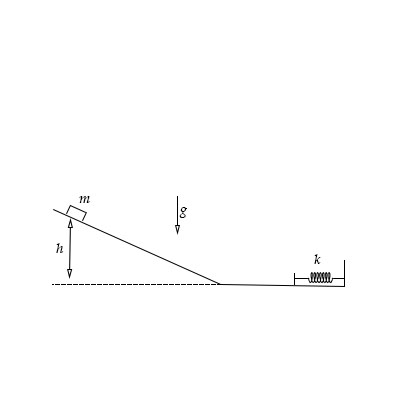# A block with mass 2.0 \ kg slides down along a frictionless inclined plane. The block starts from...

## Question:

A block with mass {eq}2.0 \ kg {/eq} slides down along a frictionless inclined plane. The block starts from rest at a height of {eq}1.8 \ m {/eq} above level ground. Ignore air resistance.

a) Find the speed of the block when it reaches the bottom of the incline.

b) Next, the block slides along frictionless level ground until it encounters a horizontal spring. The spring slows the block down to a halt momentarily. When the block stops moving, the spring compression is {eq}0.16 \ m {/eq}. Find the spring constant.## Conservation of energy

The term conservation of energy in physics can be defined as the energy from a system never increase or decrease it always be constant, it only changes its form from one system to another.

Given data:

• The mass of the block is: {eq}m = 2\;{\rm{kg}} {/eq}.
• The height from the ground is: {eq}h = 1.8\;{\rm{m}} {/eq}.

(a)

Initially the block is on the top and in the rest condition so the kinetic energy at the initial condition is zero and when the ball is released then after it reached to the ground then the potential energy of the ball is zero.

Apply conservation of energy on the block.

{eq}{P_1} + {K_1} = {P_2} + {K_2} {/eq}

Here, the initial potential energy is {eq}{P_1} {/eq}, the initial kinetic energy is {eq}{K_1} {/eq}, the final potential energy of the block is {eq}{P_2} {/eq} and the final kinetic energy of the block is {eq}{K_2} {/eq}.

Rewrite the above equation.

{eq}\begin{align*} mgh + 0 &= 0 + \dfrac{1}{2}m{v^2}\\ {v^2} &= 2gh\\ v &= \sqrt {2gh} \end{align*} {/eq}

Substitute the given values.

{eq}\begin{align*} v &= \sqrt {2 \times 9.81\;{\rm{m/}}{{\rm{s}}^2} \times 1.8\;{\rm{m}}} \\ &= 5.94\;{\rm{m/s}} \end{align*} {/eq}

Thus, the velocity of the block is {eq}5.94\;{\rm{m/s}} {/eq}.

(b)

Given:

• The compression of the spring is: {eq}x = 0.16\;{\rm{m}} {/eq}.

Apply conservation of energy on the block due to spring.

{eq}\begin{align*} {P_1} + {K_1} &= {P_2} + {K_2}\\ mgh + 0 &= \dfrac{1}{2}k{x^2} + 0\\ k &= \dfrac{{2mgh}}{{{x^2}}} \end{align*} {/eq}

Here, the stiffness of the spring is {eq}k. {/eq}

Substitute the given values.

{eq}\begin{align*} k &= \dfrac{{2 \times 2\;{\rm{kg}} \times 9.81\;{\rm{m/}}{{\rm{s}}^2} \times 1.8\;{\rm{m}}}}{{{{\left( {0.16\;{\rm{m}}} \right)}^2}}}\\ &= 2759.06\;{\rm{kg/}}{{\rm{s}}^2}\left( {\dfrac{{1\;{\rm{N}}}}{{1\;{\rm{kgm/}}{{\rm{s}}^2}}}} \right)\\ &= 2759.06\;{\rm{N/m}} \end{align*} {/eq}

Thus, the stiffness of the spring is {eq}2759.06\;{\rm{N/m}} {/eq}.